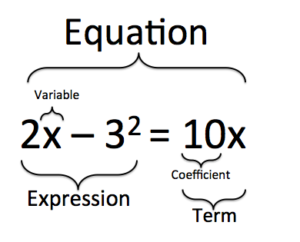## Multi-Step Equations

### Learning Outcomes

• Use properties of equality to isolate variables and solve algebraic equations
• Solve equations containing absolute valueSteps with an end in sight.

## Use Properties of Equality to Isolate Variables and Solve Algebraic Equations

First, let us define some important terminology:

• variables: variables are symbols that stand for an unknown quantity; they are often represented with letters, like x, y, or z.
• coefficient: Sometimes a variable is multiplied by a number. This number is called the coefficient of the variable. For example, the coefficient of  $3x$ is $3$.
• term: a single number, or variables and numbers connected by multiplication. $-4, 6x$ and $x^2$ are all terms.
• expression: groups of terms connected by addition and subtraction. $2x^2-5$ is an expression.
• equation: an equation is a mathematical statement that two expressions are equal. An equation will always contain an equal sign with an expression on each side. Think of an equal sign as meaning “the same as.” Some examples of equations are $y = mx +b$, $\dfrac{3}{4}\normalsize r = v^{3} - r$, and $2(6-d) + f(3 +k) =\dfrac{1}{4}\normalsize d$.

The following figure shows how coefficients, variables, terms, and expressions all come together to make equations. In the equation $2x-3^2=10x$, the variable is $x$, a coefficient is $10$, a term is $10x$, an expression is $2x-3^2$.There are some equations that you can solve in your head quickly. For example, what is the value of y in the equation $2y=6$? Chances are you didn’t need to get out a pencil and paper to calculate that $y=3$. You only needed to do one thing to get the answer: divide $6$ by $2$.

Other equations are more complicated. Solving $\displaystyle 4\left(\frac{1}{3}\normalsize t+\frac{1}{2}\normalsize\right)=6$ without writing anything down is difficult! That is because this equation contains not just a variable but also fractions and terms inside parentheses. This is a multi-step equation, one that takes several steps to solve. Although multi-step equations take more time and more operations, they can still be simplified and solved by applying basic algebraic rules.

Remember that you can think of an equation as a balance scale, with the goal being to rewrite the equation so that it is easier to solve but still balanced. The addition property of equality and the multiplication property of equality explain how you can keep the scale, or the equation, balanced. Whenever you perform an operation to one side of the equation, if you perform the same exact operation to the other side, you will keep both sides of the equation equal.

If the equation is in the form $ax+b=c$, where x is the variable, you can solve the equation as before. First “undo” the addition and subtraction and then “undo” the multiplication and division.

### Example

Solve $3y+2=11$.

### Example

Solve $3x+5x+4-x+7=88$.

Some equations may have the variable on both sides of the equal sign, as in this equation: $4x-6=2x+10$.

To solve this equation, we need to “move” one of the variable terms. This can make it difficult to decide which side to work with. It does not matter which term gets moved, $4x$ or $2x$; however, to avoid negative coefficients, you can move the smaller term.

### Examples

Solve: $4x-6=2x+10$

In this video, we show an example of solving equations that have variables on both sides of the equal sign.

## Solving Multi-Step Equations With Absolute Value

We can apply the same techniques we used for solving a one-step equation which contained absolute value to an equation that will take more than one step to solve. Let us start with an example where the first step is to write two equations, one equal to positive $26$ and one equal to negative $26$.

### Example

Solve for p. $\left|2p–4\right|=26$

In the next video, we show more examples of solving a simple absolute value equation.

Now let us look at an example where you need to do an algebraic step or two before you can write your two equations. The goal here is to get the absolute value on one side of the equation by itself. Then we can proceed as we did in the previous example.

### Example

Solve for w. $3\left|4w–1\right|–5=10$

In the two videos that follow, we show examples of how to solve an absolute value equation that requires you to isolate the absolute value first using mathematical operations.